### Home > MC1 > Chapter 5 > Lesson 5.2.3 > Problem5-64

5-64.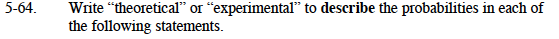For parts (a) through (e), study the Math Notes box from Lesson 5.2.2 for help understanding theoretical and experimental probability.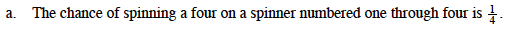This probability is based on outcomes that have the same chance of occurring. It has been calculated; it is not the result of an experiment. Can you describe it as ''theoretical'' or ''experimental"?

This probability is theoretical.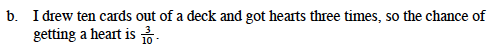This probability is the result of someone's experience or their data collected. Is this ''theoretical'' or ''experimental''?

This probability is experimental.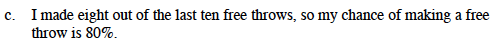Remember to refer to the Math Notes box if you need more assistance.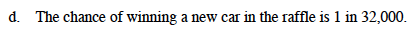This probability is theoretical.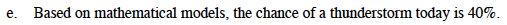Keep up the good work!